MORE IN Signals & Systems
Total marks: --
Total time: --
INSTRUCTIONS
(1) Assume appropriate data and state your reasons
(2) Marks are given to the right of every question
(3) Draw neat diagrams wherever necessary

1 (a) Give a brief classification of signals.
4 M
1 (b) Check whether the following systems are linear, causal and time invariant not $i) \ \dfrac {d^2 y(t)}{dt^2}+ 2y(t) \dfrac {dy (t)}{dt} +3ty(t)=x(t) \\ ii) \ y(n)=x^2(n)+ \dfrac{1}{x^2(n-1)}$
8 M
1 (c) Classify the following signals or energy signals or power signals:
i) x(n)=2n u(-n)
ii) x(n)=(j)n+(j)n
5 M
1 (d) A system consists of several sub-systems connected as shown in Fig. Q1 (d). Find the operator H relating x(t) to y(t) for the following sub system operators:
H1:y1(t)=x1(t) x1(t-1)
H1: y(t)=1+2x4(t)
H2: y2 (t)=|x2 (1)|
H4: y4(t)=cos (x4(t))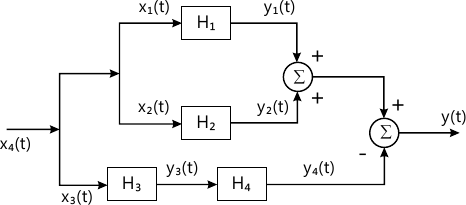3 M

2 (a) Find the continuous-time convolution integral given below:
Y(t)=cos (?t)|u(t+1)-u(t-3)|*u(t).
6 M
2 (b) Consider the i/p signal x(n) and impulse response (n) given below: $x(n)= \left\{\begin{matrix} 1, &0\le n\le 4 \\0, & otherwise \end{matrix}\right., \\ h(n)\left\{\begin{matrix}\alpha^t &0\le n \le 6. |\alpha|<1 \\0, &otherwise \end{matrix}\right.$ Obtain the convolution sum y(n)=x(n)8h(n)
8 M
2(c) Derive the following properties:
i) x(n)×h(n)=h(n)×x(n)
ii) x(n)×|h(n)×g(n)|=[x(n)×h(n)]×g(n).
6 M

3 (a) For each impulse response listed below. Determine whether the corresponding system is memoryless causal and stable;
i) h(n)=(0.99)n u(n+3)
ii) h(t)=e-31 u(t-1).
8 M
3 (b) Evaluate the step response for the LTI system represented by the following impulse response: h(t)=u(t+1)-u(t-1).
4 M
3 (c) Draw direct form I implementation of the corresponding systems: $\dfrac {d^2 y(t)}{dt^2}+ 5 \dfrac{d}{dt} y(t) + 4y(t)= x(t) + 3 \dfrac {d}{dt} x(t)$
4 M
3 (d) Determine the forced response for the system given by:
$5\dfrac {dy(t)}{dt} + 10y(t)=2x(t)$ with input x(t)=2u(t).
4 M

4 (a) State and prove time shift and periodic time convolution properties of DTFS.
6 M
4 (b) Evaluate th e DTFS representation for the signal x(n) shown in Fig. Q4(b) and sketch the spectra.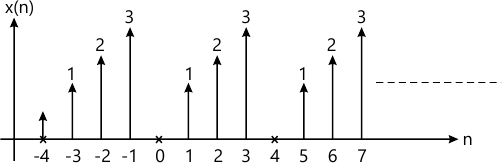8 M
4 (c) Determine the time signal corresponding to the magnitude and phase spectra shown in Fig. Q4(c), with Wo=?.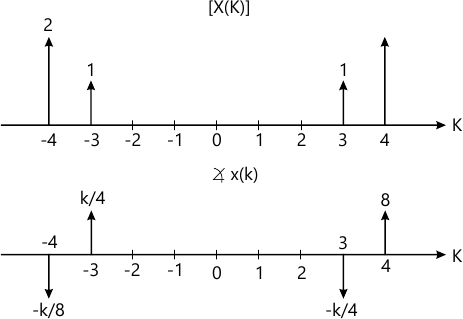6 M

5 (a) State and prove the frequency differentiation property of DTFT.
6 M
5 (b) Find the time domain signal corresponding to the DTFT shown in Fig. Q5(b).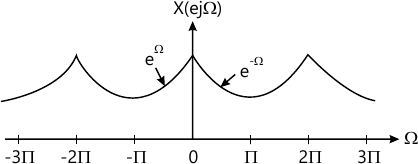5 M
5 (c) For the signal x(t) shown in Fig. Q5(c), evaluate the following quantities without explicitly computing x(w). $\displaystyle i) \ \int^{\infty}_{-\infty}x(\omega)d\omega \\ ii) \ \int^{\infty}_{- \infty}|x(\omega)|^2 d\omega \\ iii) \ \int^{\infty}_{-\infty}x(\omega)e^{j2\omega}d\omega$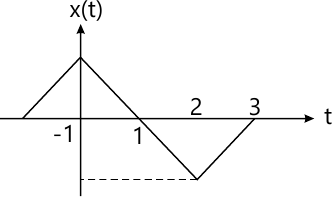9 M

6 (a) The input and output of causal LTI system are described by the differential equation $\dfrac {d^2 y(t)}{dt^2}-3 \dfrac {dy(t)}{dt}-2 y(t)=x(t)$
i) Find the frequency response of the system
ii) Find impulse response of the system
iii) What is the response of the system if x(t)=tc-1 u(t).
10 M
6 (b) Find the frequency response of the RC circuit shown in Fig. Q6(b). Also find the impulse response of the circuit.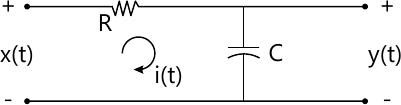10 M

7 (a) Briefly list the properties of Z-transform.
4 M
7 (b) Using appropriate properties, find the Z-transform $x(n)= n^2 \left ( \dfrac {1}{3} \right )^n u (n-2)$
6 M
7 (c) Determine the inverse Z-transform of $x(z)= \dfrac {1}{2-4 z^{-1}+2z^{-2}}$ by long division method of:
i) ROC; |z|>1.
4 M
7 (d) Determine all possible signals x(n) associated with Z-transform, $x(z)= \dfrac {\left (1/4 \right )z^{-1}}{\left [ 1-\left (1/2 \right )z^{-2} \right ] \left [ 1-\left (1/4 \right ) z^{-2} \right ]}$
6 M

8 (a) An LTI system is described by the equation
y(n)=x(n)+0.81 x(n-1)-0.81 x(n-2)-0.45 y(n-2). Determine the transfer function of the system. Sketch the poles and zeros on the Z-plane. Assess the stability.
5 M
8 (b) A system has impulse response h(n) (1/3)n n(n). Determine the transfer function. Also determine the input to the system if the output is given by: $y(n)= \dfrac {1}{2} u(n)+ \dfrac {1}{4} \left ( -\dfrac {1}{3} \right )^n u(n)$
5 M
8 (c) A linear shift invariant system is described by the difference equation. $y(n) - \dfrac {3}{4} y(n-1)+ \dfrac {1}{8} y(n-2)= x(n)+ x(n-1)$ with y(-1)=0 and y(-2)=-1
Find:
i) The natural response of the system.
ii) The forced response of the system and
iii) The frequency response of the system for a step.
10 M

More question papers from Signals & Systems